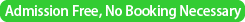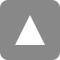﻿ Seminar talk by Prof. Paula Brito | 統計数理研究所

# Seminar talk by Prof. Paula Brito

Date&Time
2014年3月4日（火）
/ 4 March, 2014 (Tuesday)　15:00-16:00Place

/ Seminar Room 2 @ Institute of Statistical Mathematics
Speaker
Title
Conceptual Clustering of Symbolic Data Using a Quantile Representation
Abstracts

Symbolic data allow representing information with inherent varibility, thereby extending the classical tabular model, where individuals take one single value for each variable. New variable types have been introduced, allowing for multiple, possibly weighted, values for each obsevation. The main objective of Symbolic Data Analysis is to extend classical data analysis techniques to symbolic data, addressing the issues raised by the new representation spaces. As concerns clustering, methods are developed that allow clustering symbolic data, and which provide clusters directly interpreted in terms of the descriptive variables. The symbolic clustering methods presented in this talk allow considering data where each element is described by variables of possibly different types, using a bottom-up approach which merges two clusters at each step. The Quantile Representation Ichino (2008) provides a common framework to represent symbolic data described by variables of different types. It is based on the fact that a monotone property of symbolic objects is characterized by the nesting structure of the Cartesian join regions. On a discrete approach, the principle is to express the observed variable values by some predefined quantiles of the underlying distribution, which need not be equally distributed; on the other hand, variable values may be represented by the quantile function of the underlying distribution, therefore considering a continuous setup. For interval-valued variables, a distribution is assumed within each observed interval, e.g. Uniform as in Bertrand and Goupil (2000) or other; for a histogram-valued variable, quantiles of any histogram may be obtained by simply interpolation, assuming a Uniform distribution in each class (bid); for categorical multi-valued variables, quantiles are determined from the ranking defined on the categories based on their frequencies. Having a common representation setup then allows for an unified analysis of the data set, taking variables of different types simultaneously into account. An appropriate dissimilarity is used to compare data units: in the discrete approach this may be the Euclidean distance between standardized quantile vectors, whereas when using a continuous approach the Mallows distance between functions is appropriate. The proposed hierarchical/pyramidal clustering model follows a bottom-up approach; at each step, the method merges the two clusters with closest quantile representation. The newly formed cluster is then represented according to the same model, i.e., a discrete or continuous quantile representation for the new cluster is determined from the mixture of the respective distributions. This may be an uniform mixture or, alternatively, weighted by the clusters cardinalities. Notice that in the case of pyramidal clustering, the determination of the mixture weights must take into account the possible intersection between the clusters being merged. Clusters are succesively compared on the basis of the current quantile (discrete or continuous) representation. Notice that even if Uniform distibutions are assumed for the input data, the formed clusters are generally not Uniform on each variable, thus allowing for different profiles to emerge. Examples illustrate the proposed method. In a more recent work, Ichino and brito have proposed a hierarchical clustering method, which agglomerates clusters minimizing a measure, called the concept size, to achieve the compactness of the cluster descriptions. Also, the weighted self-information (WSI) based on the concept size is defined, allowing identifying informative clusters. Conjunctive logical expressions for clusters selected by the WSI are obtained. Examples show the usefulness of this hierarchical conceptual clustering method.

References:
Bertrand, P. and Goupil, F. (2000). Descriptive Statistics for Symbolic Data. In Analysis of Symbolic Data, Springer, Heidelberg, pp. 106–124.
Brito, P. and Ichino, M. (2010). Symbolic clustering based on quantile representation. In Proc. COMPSTAT 2010, Paris, France.
Brito, P. and Ichino, M. (2011). Clustering Symbolic Data Based on Quantile Representation. Workshop on Symbolic Data Analysis, Namur, Belgium.
Ichino, M. (2008). Symbolic PCA for Histogram-Valued Data. In Proc. IASC 2008, Yokohama, Japan.
Ichino, M. (2011). The quantile method for symbolic Principal Component Analysis. Statistical Analysis and Data Mining 4, 184-198, Wiley.
Irpino, A. Verde, R., (2008): Comparing Histogram Data using a Mahalanobis-Wasserstein Distance. In: Classification, Data Science and Classification, Proceeding of the Eighth Conference of the International Federation of Classification Societies (IFCS08). Springer, Berlin, 77-89.